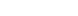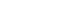# Sign Rules in Maths | Law of signs in Addition and Subtraction

First of all let’s understand what is number? Let’s see..

Number is a mathematical object used to measure, label and other mathematical operations. Basic mathematical operations are Addition, Subtraction, multiplication and division.

In this post, we will understand about rules of integers in addition and subtraction.

### Numbers Can be Positive or Negative

There are two types of numbers – Positive numbers and Negative Numbers. You can understand by the below image.

### No Sign Means Positive

If a number has no sign then you can consider it as a positive number. We usually do not put a ‘+’ sign if a number is positive. But you have to put the ‘-‘ sign if a number is negative.

For example :

• 5 is really +5.
• 9 is equal to +9.
• -7 is a -7.

I hope you now understood the difference between positive ad negative numbers. Now, let’s move ahead in the post of which are rules of sign in maths or law of signs in addition and subtraction.

### Rules of Integers in Addition

The addition of two whole numbers means you have to find the total amount of those quantities combined.

The addition is written using the plus sign  “+” between the terms. It is an infix notation. And the result is shown with an equals sign. For example,(“one plus one equals two”)(“two plus two equals four”) etc.

If signs are the same, add and keep the sign same:

Case 1: If sign of both numbers are positive, then do simple sum and keep positive sign.

For example :

(a) (+7) + (+4) = (+11)

(b) (+9) + (+2) = (+11)

(c) 5 + 7 = 12 , etc.

Case 2: If signs of both numbers are negative, then do simple sum and keep negative sign.

For example:

(d) (-2) + (-6) = (-8)

(e) (-12) + (-3) = (-15) and etc.,

If signs are different, then subtract and keep the sign of larger value.

Case 1: If the sign of the larger value is positive, then put a positive sign in the result.

For example:

(f) (-4) + (+15) = (+11)

(g) (+9) + (-3) = (6)

Case 2: If the sign of the larger value is negative, then put a negative sign in the result.

For example:

(f) (+4) + (-15) = (-11)

(g) (-9) + (+3) = (-6)

### Rules of Integers in Subtraction

Subtraction is the operation of removing objects from a collection. It is written using the minus sign  “-” between the terms. And the result is expressed with an equals sign. For example,

5 – 3 = 2 (“five minus three equals two”)

7 – 3 = 4 (“seven minus three equals four”) and etc.

Subtraction Sign Rules :

” Change the sign of the number which is needed to be subtracted then, follow Addition Sign Rules “

You will be able to understand this by below example :

Ex. 1 : (-13) – (+7)

= (-13) + (-7) = (-21) [Changed the sign from (+7) to (-7), then followed addition sign rule]

Ex 2: (-5) – (-9)

= (-5) + (+9) = (+4) [Changed the sign from (-9) to (+9), then followed addition sign rule]

Ex 4: (+7) – (-6)

= (+7) + (+6) = (+13) [Changed the sign from (-6) to (+6), then followed addition sign rule]

Ex 5: (+12) – (+4)

= (+12) + (-4) = (+8) [Changed the sign from (+4) to (-4), then followed addition sign rule]

That’s all. Now you know all the laws of signs in addition and subtraction.

If you have any queries or suggestions for us then you can just comment down below and let us know about those. Our team will respond to you within 24 hours.

Hope you like this post on which are rules of sign in maths? To get more knowledge about business stay tuned with us. Don’t forget to follow our all social media handles.